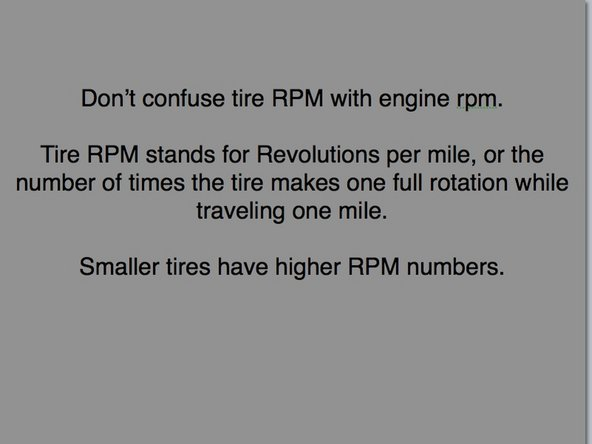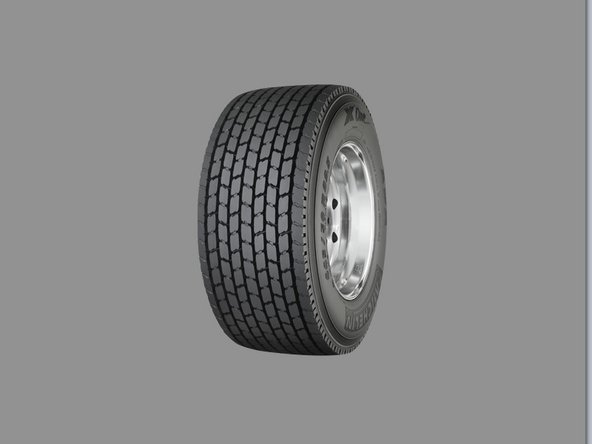1. ## Step 1 How to Calculate Your Rear End Ratio• Here is the formula to calculate rear end ratio:

• =(60*RPM)/(Tire Size*Xmission Final Ratio*Road Speed)

• Here's what you need to know:

• Your Tire size in a number represented as "revolutions per mile"

• All tires can be slightly different and you would have to look up your specific tire make and model to know the true number, but most of the time I use generic numbers and that will get you close. In the next step I'll show you the numbers I use for each tire size.

2. ## Step 2• Here are the numbers I use for the different tire sizes

• 11R (Tall) 24.5 = 473 revolutions per mile

• Low Pro 24.5 = 496 revolutions per mile

• 11R (Tall) 22.5 = 496 revolutions per mile

• Low Pro 22.5 = 512 revolutions per mile

• MIchelin 445 Wide Single = 527 revolutions per mile

3. ## Step 3• Then you also need to know your transmission final ratio.

• Transmission Final Ratios that I use:

• Direct drive = 1

• Single OverDrive = .85

• Double OverDrive = .73

• ** These numbers may not be exact, but they are usually close enough for the calculation, MOST transmissions will be a double overdrive, and will use a .73 final ratio for the calculation.

4. ## Step 4• Now take your engine RPM at a given road speed and you'll have all 4 numbers you need for the calculation, lets use:

• 1325 RPM at 60MPH

• Low Pro 22.5 (512)

• Double Overdrive 13 speed (.73)

• ** Don't confuse the first number 60 with our road speed, the first number in the equation is ALWAYS 60

• Do the math and you'll see that your rear end ratio is 3.55

5. ## Step 5Finish Line

13 other people completed this guide.

### Author

#### Kevin

Member since: 11/02/2011

6,026 Reputation

3 Guides authored

### One Comment

That was great. I am going to check the tach at 60mph later...but from what I can remember, it looks like our rear ends are 2.63 based on rpm's of 1350 on a direct transmission 10 speed and 22.5 lo-pro tires. But I will double check that 1350 number. This could be the reason our truck is strong but won't pull the hills. It's a "rollscanhardly" for sure. Thanks for the help.